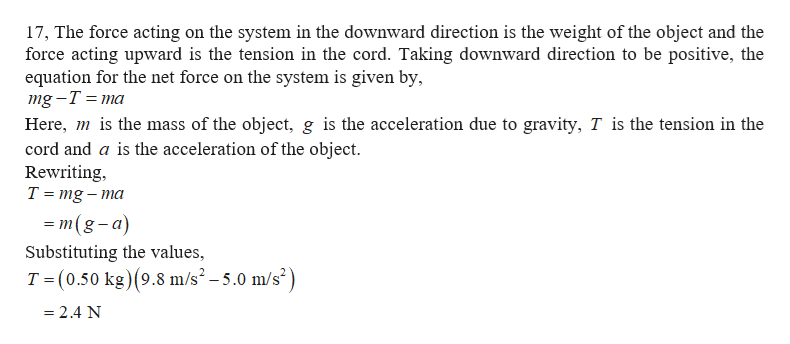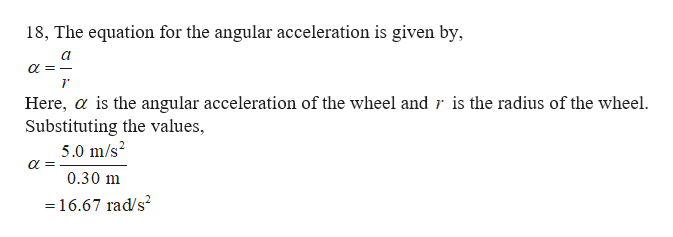# Ringtone15. find the linear acceleration of the sphere, in m/sec216. for the sphere to make pure rotation, find the magnitude of the static frictional coefficient u ?A wheel (radius = 0.30 m) is mounted on a frictionless, horizontal axis. A light cord wrapped aroundthe wheel supports a 0.50-kg object. When released from rest the object falls with a downwardacceleration of 5.0 m/sec217. Find the tension on the cord.18. Find the angular acceleration of the wheelT19. Find the moment of inertia of the wheelmgA solid disk (M = 10 kg and R = 70 cm) is rotating at 300 rpm initially. Then a smaller ring ( m = 3.0kg and r 40 cm ) is dropped concentrically onto the disk and they rotate together with a new angularvelocity. (Hint: the rotational inertia of the small ring is mr, not /2 mr2!)20. find the final angular velocity of the system in rpm.Y21. find the change in rotational kinetic energy of the system.M22. find the percent of energy loss during this collision between the solid disk and the ringA hollow spherical ball is let go from the top of a track and goes around a circular loop (R = 60 cm ).23. What must be the minimum height of the track so that the ball will not fall off the track at thetopmost point of the loop?R24. When the above condition is met, what is the tangential speed of the ball when it reaches thetopmost point?A 25-ft crane supported at its lower end by a pin is elevated by a horizontal cable. A 250-lb load issuspended through the cable. The center of gravity of the crane is 10 ft from the pin, and the craneweighs 200 lb. Hint: You don't have to convert the unit since they cancel out on both sides.25. Find the tension in the cable26. Find the total force acting on the pin.60250bW(260ib)לר

Question
1 views

17 18 19help_outlineImage TranscriptioncloseRingtone 15. find the linear acceleration of the sphere, in m/sec2 16. for the sphere to make pure rotation, find the magnitude of the static frictional coefficient u ? A wheel (radius = 0.30 m) is mounted on a frictionless, horizontal axis. A light cord wrapped around the wheel supports a 0.50-kg object. When released from rest the object falls with a downward acceleration of 5.0 m/sec2 17. Find the tension on the cord. 18. Find the angular acceleration of the wheel T 19. Find the moment of inertia of the wheel mg A solid disk (M = 10 kg and R = 70 cm) is rotating at 300 rpm initially. Then a smaller ring ( m = 3.0 kg and r 40 cm ) is dropped concentrically onto the disk and they rotate together with a new angular velocity. (Hint: the rotational inertia of the small ring is mr, not /2 mr2!) 20. find the final angular velocity of the system in rpm. Y 21. find the change in rotational kinetic energy of the system. M 22. find the percent of energy loss during this collision between the solid disk and the ring A hollow spherical ball is let go from the top of a track and goes around a circular loop (R = 60 cm ). 23. What must be the minimum height of the track so that the ball will not fall off the track at the topmost point of the loop? R 24. When the above condition is met, what is the tangential speed of the ball when it reaches the topmost point? A 25-ft crane supported at its lower end by a pin is elevated by a horizontal cable. A 250-lb load is suspended through the cable. The center of gravity of the crane is 10 ft from the pin, and the crane weighs 200 lb. Hint: You don't have to convert the unit since they cancel out on both sides. 25. Find the tension in the cable 26. Find the total force acting on the pin. 60 250b W(260ib) לר fullscreen
check_circle

Step 1help_outlineImage Transcriptionclose17, The force acting on the system in the downward direction is the weight of the object and the force acting upward is the tension in the cord. Taking downward direction to be positive, the equation for the net force on the system is given by, mg -T %3D тa Here, mis the mass of the object, g is the acceleration due to gravity, T is the tension in the cord and a is the acceleration of the object Rewriting T mg-ma - т(g-а) Substituting the values, T (0.50 kg) (9.8 m/s2 -5.0 m/s2) - 2.4 N fullscreen
Step 2help_outlineImage Transcriptionclose18, The equation for the angular acceleration is given by Here, a is the angular acceleration of the wheel and r is the radius of the wheel Substituting the values, 5.0 m/s2 0.30 m 16.67 rad/s2 fullscreen

### Want to see the full answer?

See Solution

#### Want to see this answer and more?

Solutions are written by subject experts who are available 24/7. Questions are typically answered within 1 hour.*

See Solution
*Response times may vary by subject and question.
Tagged in

### Kinematics